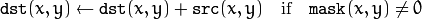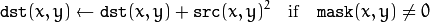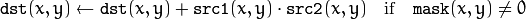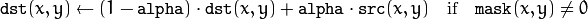Motion Analysis and Object Tracking¶

accumulate¶

Adds an image to the accumulator.

C++: void accumulate(InputArray src, InputOutputArray dst, InputArray mask=noArray() )
Python: cv2.accumulate(src, dst[, mask]) → None
C: void cvAcc(const CvArr* src, CvArr* dst, const CvArr* mask=NULL )
Python: cv.Acc(src, dst, mask=None) → None
Parameters: src – Input image as 1- or 3-channel, 8-bit or 32-bit floating point. dst – Accumulator image with the same number of channels as input image, 32-bit or 64-bit floating-point. mask – Optional operation mask.

The function adds src or some of its elements to dst :The function supports multi-channel images. Each channel is processed independently.

The functions accumulate* can be used, for example, to collect statistics of a scene background viewed by a still camera and for the further foreground-background segmentation.

accumulateSquare¶

Adds the square of a source image to the accumulator.

C++: void accumulateSquare(InputArray src, InputOutputArray dst, InputArray mask=noArray() )
Python: cv2.accumulateSquare(src, dst[, mask]) → None
C: void cvSquareAcc(const CvArr* src, CvArr* dst, const CvArr* mask=NULL )
Python: cv.SquareAcc(src, dst, mask=None) → None
Parameters: src – Input image as 1- or 3-channel, 8-bit or 32-bit floating point. dst – Accumulator image with the same number of channels as input image, 32-bit or 64-bit floating-point. mask – Optional operation mask.

The function adds the input image src or its selected region, raised to a power of 2, to the accumulator dst :The function supports multi-channel images. Each channel is processed independently.

accumulateProduct¶

Adds the per-element product of two input images to the accumulator.

C++: void accumulateProduct(InputArray src1, InputArray src2, InputOutputArray dst, InputArray mask=noArray() )
Python: cv2.accumulateProduct(src1, src2, dst[, mask]) → None
C: void cvMultiplyAcc(const CvArr* src1, const CvArr* src2, CvArr* dst, const CvArr* mask=NULL )
Python: cv.MultiplyAcc(src1, src2, dst, mask=None) → None
Parameters: src1 – First input image, 1- or 3-channel, 8-bit or 32-bit floating point. src2 – Second input image of the same type and the same size as src1 . dst – Accumulator with the same number of channels as input images, 32-bit or 64-bit floating-point. mask – Optional operation mask.

The function adds the product of two images or their selected regions to the accumulator dst :The function supports multi-channel images. Each channel is processed independently.

accumulateWeighted¶

C++: void accumulateWeighted(InputArray src, InputOutputArray dst, double alpha, InputArray mask=noArray() )
Python: cv2.accumulateWeighted(src, dst, alpha[, mask]) → None
C: void cvRunningAvg(const CvArr* src, CvArr* dst, double alpha, const CvArr* mask=NULL )
Python: cv.RunningAvg(src, dst, alpha, mask=None) → None
Parameters: src – Input image as 1- or 3-channel, 8-bit or 32-bit floating point. dst – Accumulator image with the same number of channels as input image, 32-bit or 64-bit floating-point. alpha – Weight of the input image. mask – Optional operation mask.

The function calculates the weighted sum of the input image src and the accumulator dst so that dst becomes a running average of a frame sequence:That is, alpha regulates the update speed (how fast the accumulator “forgets” about earlier images). The function supports multi-channel images. Each channel is processed independently.